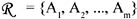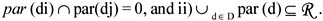# FOLD

This operator was proposed in Gyssens & Lakshamanan (1997). They first define an n-dimensional aggregate data (table) schema S as a triple <D, R, par>, where D = {d1, d2, , dn} is a set of dimension names,is a set of attributes and par: D 2{A1, A2, , Am}, such that: i) for all i, j = 1, , n, with i j,The authors denote par (di) by Xi. The definition of the fold operator is:

Let s be a MAD with schema S and let d be one of the dimensions of D.

We define foldd(s) as a MAD with schema S' = <D \ {d}, R, par'>, where, for all di in D \ {d}, par' (di) = par (di), and with an instance tab S'(rep(s)), where rep(s) is the denotation of the relational representation f(s) of the table instance s, i.e., a relation with schema R.Multidimensional Databases: Problems and Solutions
ISBN: 1591400538
EAN: 2147483647
Year: 2003
Pages: 150